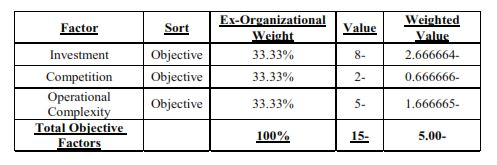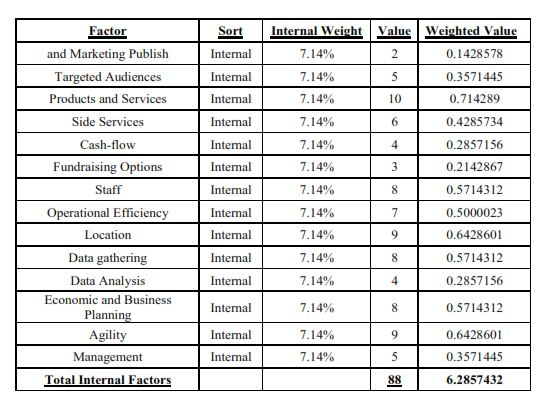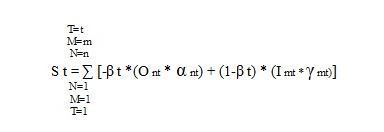# Cowley’s Model for predicting a business success

## introduction

The article presents an intuitive business model based on the concept of the entrepreneur and/or business or economic consultant. Sometimes objective market conditions prevent us from realizing a business idea on the one hand and internal capabilities on the other hand, that may create difficulties. Although a feasibility study is usually carried out through a business or economic plan, the proposed model provides a quick way to conduct regular checks and prepare business analysis as of the test date. The model simplifies the decision-making process and strives for intra-organizational perfection with the objective ones. It should be noted that certain variables can be both objective and intra-organizational.

## Objective Factors – Affecting Business Success *

Objective factors are given factors for the entrepreneurs that need to take them as facts. For example: taxation, levies, price of equipment, inventory prices, competitors, rivalry in the industry, operational complexity, etc. An objective factor’s sign is negative. In other words, the higher its absolute value, the more it affects and threatens the business. The following table is an example of how objective factors and their values are recorded according to the extent of their impact.

The relative weight of the factors is a matter of ranking according to the decision of the developer or the consultants. For simplicity, it was assumed that their weight was the same. The values of each factor are between 0 and 10 (negative sign).
There may be additional objective factors that will be recorded according to the type of business and its characteristics and as long as the entrepreneur has no control over them. The following table is an example only for explanation of the model.## Intra-organizational factors affecting business success *

Organizational variables include operating efficiency, cash flow, interest rate, ability to raise capital and investments, ability to target audiences, advertising and marketing, staff, location, products and related services, management and control.
Usually at the first stage, these factors receive a relatively low score based on the entrepreneur’s assessments, but later each factor will receive a higher value according to strategic and business planning and the manner of coping. Each variable has its internal weight. Additional factors may be added according to the type of business, but the principles are the same. The values of each factor are between 0 and 10 (positive sign). For simplicity, we assume that the relative weight of each variable is the same.
The computational result of the influence of the objective and intra-organizational factors is 1.1667> 0.

* (50% in the final equation – or in any ratio deemed appropriate)

##Example 1

Let us assume that the total relative weight of the objective variables is 5 (negative sign) while the value of the internal factors is +10 because of striving by monitoring and improving of all organizational variables that we noted. In such a case, the final score will be as follows:

50% * (-5) + 50% * (10) = 2.5. Since we have received a positive value, we may tend to work towards financing this entrepreneurship or at least to compare it with other projects and choose the more virtuous ones. The odds value according to the conversion formula (below) is 62.5%

## Example 2

Let us assume that the total value of the weighted objective variables is 5 (negative sign) while the value of the internal factors is +5 (positive sign). In this case, we get a value of 0 and therefore we are indifferent or alternatively, we need to add relevant variables or improve existing ones in order to gain a better score. The odds value according to the conversion formula is 50%

## Example 3

Suppose that the total value of the weighted objective variables is -8 (a negative sign), whereas the value of the internal factors is +5 (in a positive sign). In this case, we get the final value of -1.5 (negative sign). This means that the objective variables are stronger than the internal performance ability, and therefore the level of risk is too high, unless Intra-organizational variables scores are improved by working with business and economic consultants, the odds can’t allow us to proceed. The odds value according to the conversion formula is only 42.5% (less than 50%)

## In conclusion

This model, though intuitive, may be a sophisticated tool for a skilled user to compare actual performance to what is assessed in the business plan, to constantly improve intra-organizational factors, and to examine them from time to time. Business owners or business consultants will be able to examine and determine at a high level of probability, the degree of expected odds or the level of risk with which they are willing to deal. The following is a generic formula of the model:St – The test result at a given point in time. In the case of a temporal series we obtain T = 1 to T = T, the relative weights will be a function of the dependence between the objective variables and the variables within organizations through different kinds of expressions, and not necessarily Linear. An autonomous factor A can be added to the equation in the case of time series and random variables.
O – is a given objective variable subject to the following conditions: O> = -10 <= 0
α – is the relative weight of objective factor N where α is from 1 to n and sums up to Σα = 1 at a certain observation or test
β – is the relative weight between the objective factors and the internal factors, under the condition of – β> 0 and less than 1
I – is a variable within a given organizational condition:> = 0 and <= 10
γ – is the relative weight of the intra-organizational factor when γ is from 1 to m and sums to = 1
N – the number of objective variables
M – Number of intra-organizational variables
T-Test date. You can build time series and look for the ratio between them and find their weights or functions, linear and/or non-linear regression.

The conversion formula of the calculation result of the success rate is as follows:

## Final Odds – P = (10 + St) / 20

Where P is the success rate and St, is a result of the calculation according to the formula listed above.